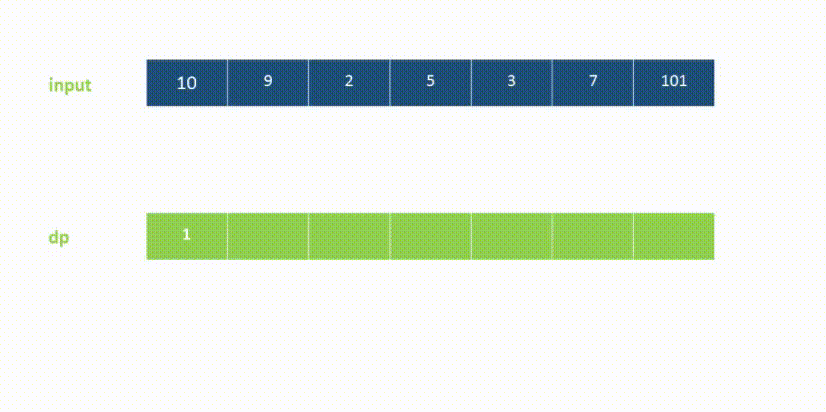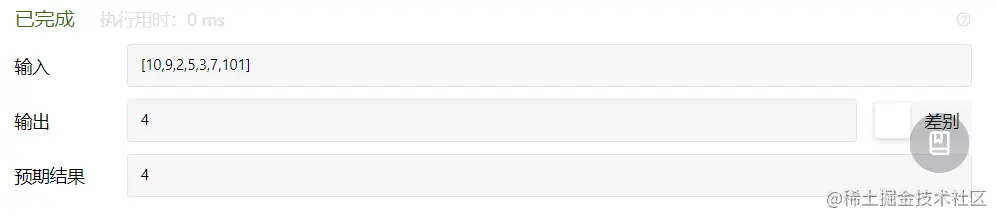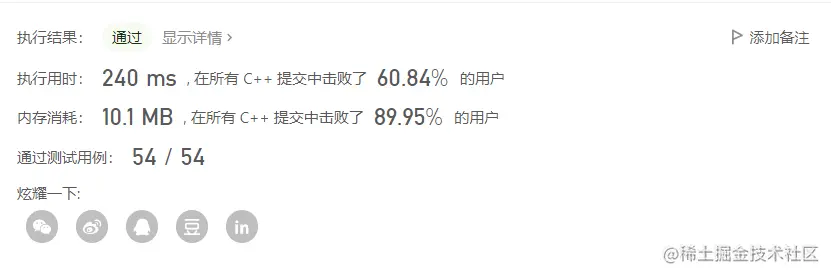# 算法题每日一练---第70天：最长递增子序列

2888

## 二、题目要求

### 样例 1

``````输入： nums = [10,9,2,5,3,7,101]

``````

### 样例 2

``````输入： nums = [0,1,0,3,2,3]

``````

### 考察

``````1.子序列、动态规划
2.建议用时15~35min
``````

## 三、问题分析

### 第三步 规律归纳：`定义j=i-1`

dp[i]=dp[j]+1;(加入的这个1就是nums[i])。

`dp[i]=max(dp[j]+1,dp[i]);`

## 四、编码实现

``````class Solution {
public:
int lengthOfLIS(vector<int>& nums) {
int i,j,n=nums.size(),dp[n+2],ans=0;//初始化变量
for(i=0;i<n;i++)//循环i
{
dp[i]=1;//变量初始
for(j=0;j<i;j++)//遍历i之前的数字
{
if(nums[j]<nums[i])//如果i比j对应的数据大
dp[i]=max(dp[j]+1,dp[i]);//nums[i]加入大家庭
}
ans=max(ans,dp[i]);//寻找最大值
}
return ans;//返回结果
}
};
``````

## 五、测试结果•••••••••